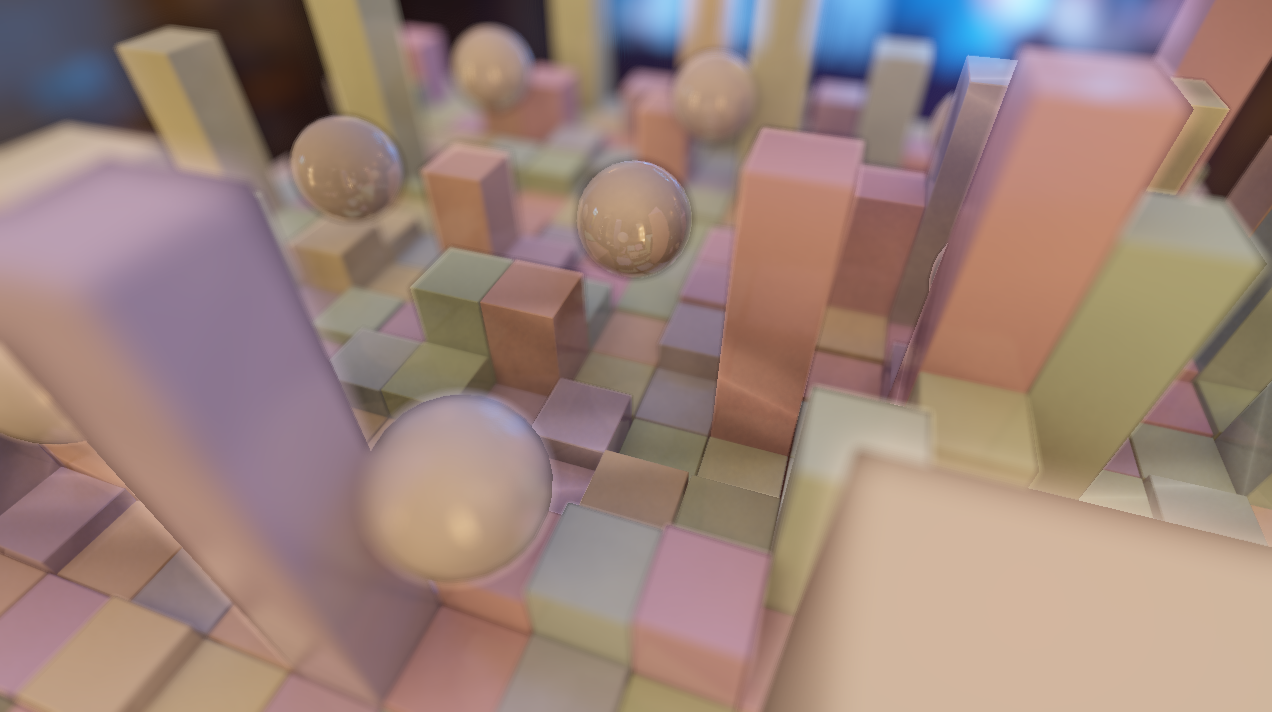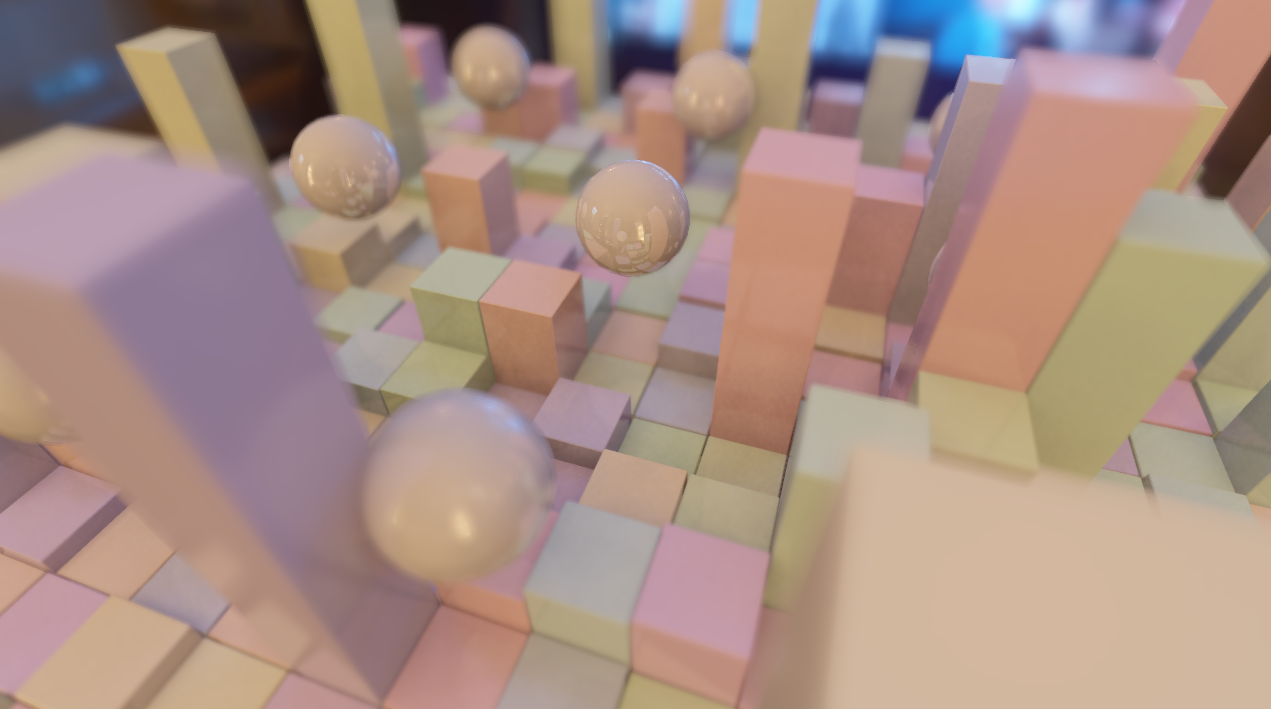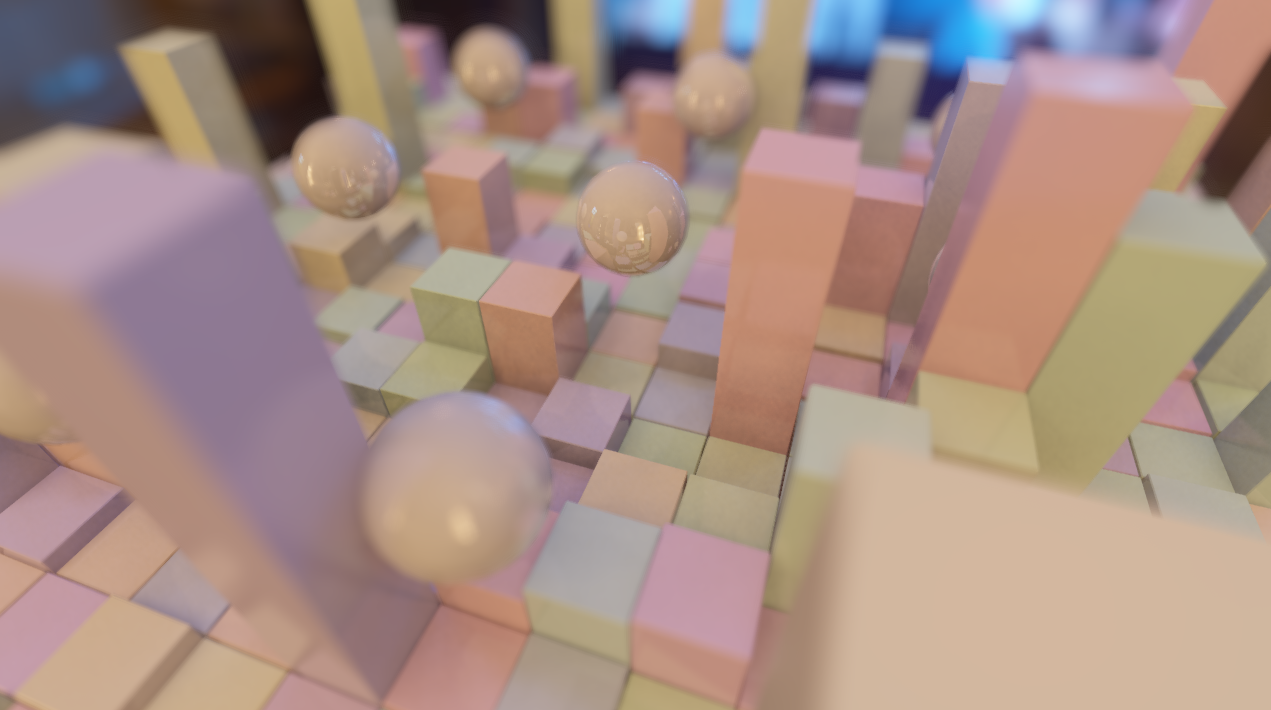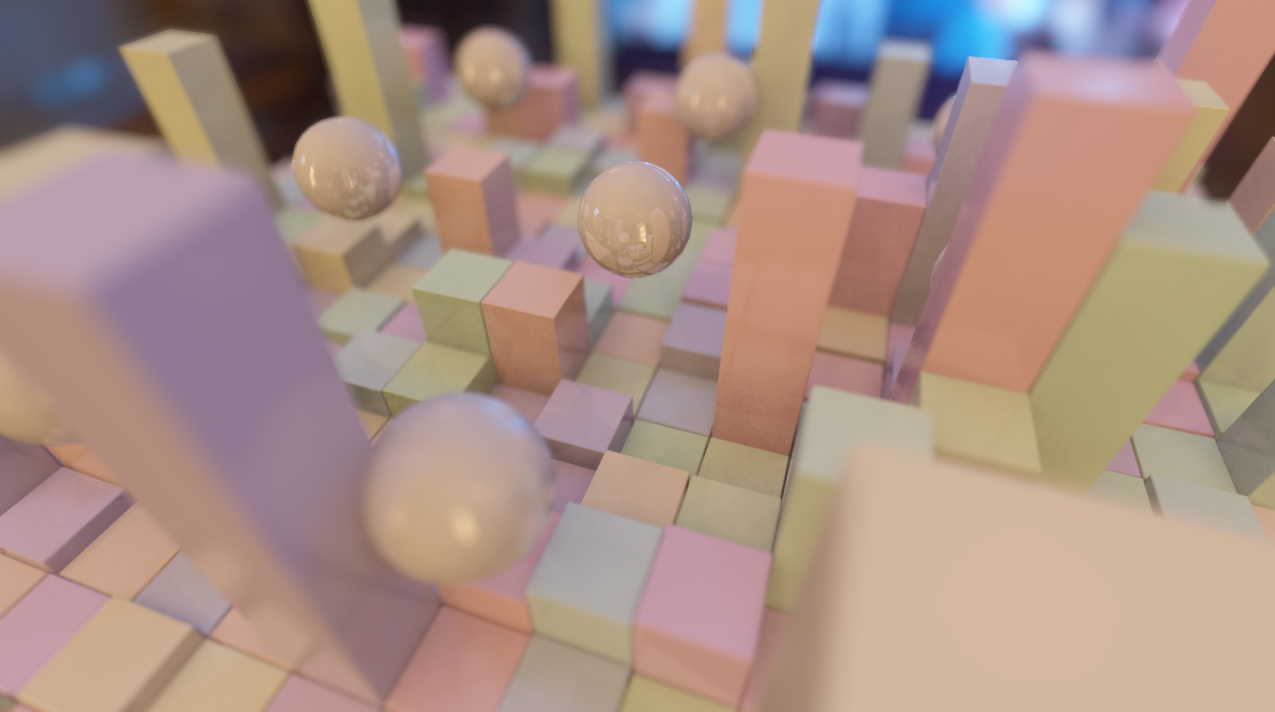When I implemented bokeh depth of field I stumbled upon a neat blending trick almost by accident. In my opinion, the quality of depth of field is more related to how objects of different depths blend together, rather than the blur itself. Sure, bokeh is nicer than gaussian, but if the blending is off the whole thing falls flat. There seems to be many different approaches to this out there, most of them requiring multiple passes and sometimes separation of what’s behind and in front of the focal plane. I experimented a bit and stumbled upon a nice trick, almost by accident.

I’m not going to get into technical details about lenses, circle of confusion, etc. It has been described very well many times before, so I’m just going to assume you know the basics. I can try to summarize what we want to do in one sentence – render each pixel as a discs where the radius is determined by how out of focus it is, also taking depth into consideration “somehow”.

Taking depth into consideration is the hard part. Before we even start thinking about how that can be done, let’s remind ourselves that there is no correct solution to this problem. We simply do not have enough information. Real depth of field needs to know what’s behind objects. In a post processing pass we don’t know that, so whatever we come up with will be an approximation.

I use a technique sometimes referred to as scatter-as-gather. I like that term, because it’s very descriptive. GPU’s are excellent at reading data from multiple inputs, but hopelessly miserable at writing to multiple outputs. So instead of writing out a disc for each pixel, which every sane programmer would do in a classic programming model, we have to do the whole operation backwards and compute the sum of all contributing discs surrounding a pixel instead. This requires us to look as far out as there can be any contributions and the safest such distance is the maximum disc radius. Hence, every pixel becomes the worst case. Sounds expensive? It is! There are ways to optimize it, but more on that later. Here is the test scene before and after blur with a fixed disc size for all pixels:Now to the hard part. When gathering discs, each one of them have different sizes. That in itself it not very hard, just compare the distance to the disc radius. If it’s smaller it contributes, otherwise not. The hard part is how it should contribute and when. Taking a stab at a “correct” solution, I compute how much a sample from each disc contributes. A larger disc means that the pixel intensity will get spread out over a larger area, so each individual sample gives a smaller contribution. Doing the math right, summing up everything in the end should yield the same overall intensity in the image. This is what it looks like:It’s not totally wrong, but it’s definitely not right. Foreground and background objects do get blurred, but foreground objects get darkened around edges. You might also notice bright streaks across the focus areas. The streaks are artefacts from working with discrete samples in something that should be continuous. It’s hard to do the correct thing when the disc size approaches zero, since you have to take at least one sample. What about the dark edges? If we did this the right way, we would actually blend in each disc, allowing some of the background to shine through. Since that information is occluded this is what we get.

Instead of trying to compute the correct intensity from each disc sample, let’s just sum them up and remember the total, then divide with the total afterwards. This is pretty classic conditional blur and always gives the correct intensity:

``````for each sample
if sample contributes then
color += sample * contribution
total += contribution
end
end
color /= total
``````Definitely better. Both the streaks and the dark corners are gone, but foreground objects don’t blur correctly over a sharp background. This is because the contribution from in-focus objects have a stronger weight than the large discs in the foreground. There are several ways to attack this issue. One that I found to look relatively good is to compute the contribution using the distance to the sample instead of the disc size. This is far from correct, but gives a nicer fall-off:Now to the trick. Instead of computing a contribution based on disc size or distance, I use a fixed weight for all contributing samples no matter the distance or disc size, and blend in the current average if there is no contribution:

``````color = centerColor
total = 1.0
for each sample
if sample contributes then
color += sample
else
color += color/total
end
total += 1.0
nextcolor /= total
``````

Huh? What this does is basically to give each contributing sample equal weight, but when there is no contribution, the current average is allowed to grow stronger and get more impact on the result. This is what it looks like:Not bad. The blur is continuous and nice for both foreground and background objects. Note that this only works when sampling in a spiral, starting at the center pixel being shaded, because the first sample needs to be the pixel itself. I’m not going to try and explain details on why this algorithm works, simply because I’m not sure. I found it by accident, and I have spent days trying other methods but nothing works as well as this one.

There is one small thing to add before this is usable. The background shouldn’t blur over foreground object that are in focus. Note though that if the foreground is out of focus, we want some of the background to blur in with it. What I do is to clamp the background circle of confusion (disc size) between zero and the circle of confusion of the center pixel. This means that the background can contribute only up to the amount of blurryness of the pixel being shaded. The scatter-as-gather way of thinking requires a lot of strong coffee.

``````if sample depth > center depth then
sample size = clamp(sample size, 0, center size)
end
``````

Here is what the final image looks like:A couple of notes on my implementation. After some experimentation I changed the clamping of sample size to `clamp(sample size, 0, center size*2.0)`. For larger max radius I increase it even more. This controls how much of the background gets blended into a blurry foreground. It is totally unphysical and is only there to approximate the occluded information behind the foreground object.

The following code is written for clarity rather than speed. My actual implementation is in two passes, calculating the circle of confusion for each pixel and stores in the alpha component, at the same time downscaling the image to quarter resolution (half width, half height). When computing the depth of field I also track the average sample size and store in the alpha channel, then use this to determine wether the original or the the downscaled blurred version should be used when compositing. More on performance optimizations in a future post.

``````uniform sampler2D uTexture; //Image to be processed
uniform sampler2D uDepth; //Linear depth, where 1.0 == far plane
uniform vec2 uPixelSize; //The size of a pixel: vec2(1.0/width, 1.0/height)
uniform float uFar; // Far plane

const float GOLDEN_ANGLE = 2.39996323;
const float MAX_BLUR_SIZE = 20.0;
const float RAD_SCALE = 0.5; // Smaller = nicer blur, larger = faster

float getBlurSize(float depth, float focusPoint, float focusScale)
{
float coc = clamp((1.0 / focusPoint - 1.0 / depth)*focusScale, -1.0, 1.0);
return abs(coc) * MAX_BLUR_SIZE;
}

vec3 depthOfField(vec2 texCoord, float focusPoint, float focusScale)
{
float centerDepth = texture(uDepth, texCoord).r * uFar;
float centerSize = getBlurSize(centerDepth, focusPoint, focusScale);
vec3 color = texture(uTexture, vTexCoord).rgb;
float tot = 1.0;
for (float ang = 0.0; radius<MAX_BLUR_SIZE; ang += GOLDEN_ANGLE)
{
vec2 tc = texCoord + vec2(cos(ang), sin(ang)) * uPixelSize * radius;
vec3 sampleColor = texture(uTexture, tc).rgb;
float sampleDepth = texture(uDepth, tc).r * uFar;
float sampleSize = getBlurSize(sampleDepth, focusPoint, focusScale);
if (sampleDepth > centerDepth)
sampleSize = clamp(sampleSize, 0.0, centerSize*2.0);